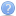Customize position of labels in Polar chart
Any progress on this issue?OxyPlot: AngleAxis labels rotation in Polar Plotspolar plot - which series to use?
Can you draw (in MS paint etc.) what's your goal?

Just to clarify...polar plot - which series to use?
Sample code: ..\Source\Examples\ExampleLibrary\Discussions\DiscussionExamples.cs

[Example("#549839: Polar plot with custom arrow series")]
public static PlotModel PolarPlotWithArrows()
{
var model = new PlotModel { Title = "Custom arrow series", PlotType = PlotType.Polar, PlotAreaBorderColor = OxyColors.Undefined };
model.Axes.Add(new AngleAxis { Minimum = 0, Maximum = 360, MajorStep = 30, MinorStep = 30, MajorGridlineStyle = LineStyle.Dash });
model.Axes.Add(new MagnitudeAxis { Minimum = 0, Maximum = 5, MajorStep = 1, MinorStep = 1, Angle = 90, MajorGridlineStyle = LineStyle.Dash });
model.Series.Add(new ArrowSeries549839 { EndPoint = new DataPoint(1, 40) });
model.Series.Add(new ArrowSeries549839 { EndPoint = new DataPoint(2, 75) });
model.Series.Add(new ArrowSeries549839 { EndPoint = new DataPoint(3, 110) });
model.Series.Add(new ArrowSeries549839 { EndPoint = new DataPoint(4, 140) });
model.Series.Add(new ArrowSeries549839 { EndPoint = new DataPoint(5, 180) });
return model;
}

private class ArrowSeries549839 : XYAxisSeries
{
private OxyColor defaultColor;

public ArrowSeries549839()
{
this.Color = OxyColors.Automatic;
this.StrokeThickness = 2;
}

public DataPoint StartPoint { get; set; }

public DataPoint EndPoint { get; set; }

public OxyColor Color { get; set; }

public double StrokeThickness { get; set; }

protected override void SetDefaultValues(PlotModel model)
{
if (this.Color.IsAutomatic())
{
this.defaultColor = model.GetDefaultColor();
}
}

public OxyColor ActualColor
{
get
{
return this.Color.GetActualColor(this.defaultColor);
}
}

public override void Render(IRenderContext rc, PlotModel model)
{
// transform to screen coordinates
var p0 = this.Transform(this.StartPoint);
var p1 = this.Transform(this.EndPoint);

var direction = p1 - p0;
var normal = new ScreenVector(direction.Y, -direction.X);

// the end points of the arrow head, scaled by length of arrow
var p2 = p1 - (direction * 0.2) + (normal * 0.1);
var p3 = p1 - (direction * 0.2) - (normal * 0.1);

// draw the line segments
rc.DrawLineSegments(new[] { p0, p1, p1, p2, p1, p3 }, this.ActualColor, this.StrokeThickness);
}
}polar plot - which series to use?
You have to use XYAxisSeries.

Therefore you need to inherit from this class.

CustomXYAxisSeries plotItem = new CustomXYAxisSeries ();
plotItem.EndPoint = new DataPoint(0, 23);

Let me know if it works...polar plot - which series to use?
Hi,

Can you post some sample code?Customize position of labels in Polar chartOxyPlot : integration in website/webservice
I've got it working!

Big thanks!OxyPlot : integration in website/webservice
Is it possible to build the OxyPlot.WindowsForms.dll in .NET 3.5 ?
﻿